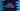# Find out the multiplication of two numbers in Python# Find out the multiplication of two numbers in Python :

Calculating the multiplication is a basic arithmetic operation. Almost in all programming language, the multiplication process is the same. In this tutorial, we will learn how to find out the multiplication of two numbers in python.

This is a beginner level python programming tutorial and you will learn how to read user inputs, how to convert user input to an integer, how to calculate the multiplication of two numbers and how to print out a value.

The program will ask the user to enter the numbers and then it will calculate the multiplication of the numbers. Finally, it will print out the multiplication result. Note that, we don’t need any extra modules to find out the multiplication of two numbers.

Character ‘*’ is used to find out the multiplication of two numbers. If we are using ‘a * b’, it will print the multiplication of ‘a’ and ‘b’. If you want to calculate the multiplication of ’n’ numbers, not only two, we can do it in a chain. For example, if we want to find out the multiplication of ‘a’,’b’,’c’, ’d’ and ‘e’, we can find out the multiplication as ‘a * b * c * d * e’.

## Python program :

Below is the python program for the above problem :

``````first_number = int(input("Enter your first number : "))
second_number = int(input("Enter your second number : "))

final_result = first_number * second_number

print("Multiplication of {} and {} is : {}".format(first_number,second_number,final_result))``````

### Explanation :

The commented numbers in the above program denote the step numbers below :

1. Ask the user to enter the first and second number. Read it and store it in firstnumber_ and secondnumber_ variables. Here, we are using the input() method to take the user input. Also, we are converting the input to an integer using the_ int()_ method. Because ‘input()’ method reads the value as a string. We need to convert it to an integer value for calculating the multiplication. Wrapping it inside the int() method converts a string value to its integer representation.For example, if the user will enter an integer value like ’12’, ’13’ etc., it will work. But it will fail for any other string values. Because,’12’,’13’ etc. can be converted to integer but other string values can’t be converted. So, it will throw one error.This error is ‘ValueError’. Note that these errors are different for different scenarios. For this example, we will get one ValueError if any non-integer values are entered.

If the user will enter anything else than an integer, e.g. a string, it will throw one ValueError

1. _Next, find out the multiplication of these numbers. _* is the multiplication operator in python as mentioned above. It will calculate the multiplication of ‘firstnumber’ and ‘secondnumber’ variables and return the multiplication result. We are storing the result in the ‘finalresult’ variable. Instead of storing the multiplication value in a different variable, we can also print the multiplication result directly using a ‘print_’ statement.
2. Finally, print out the result to the user. Note how we are using the curly braces to print out the results. ‘.format()’ is the preferred way to print out any variables in a function.

### Sample Output :

You can see that if the input is ‘ff’, it can’t convert to an integer and one ‘ValueError’ is raised.

## Conclusion :

In this tutorial, we have learned how to find out the multiplication of two numbers in python. Try to run the above program on your machine and drop one comment below if you have any queries.